### Home > CALC > Chapter 6 > Lesson 6.1.1 > Problem6-11

6-11.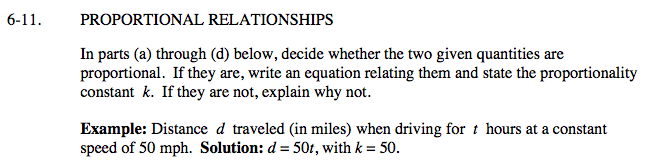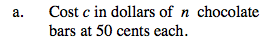c and n are proportional
c = 0.50n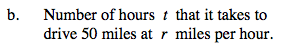distance = (rate)(time)
Is rate proportional to time?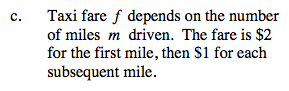Write an equation for the total taxi fare.
Is this a proportional relationship?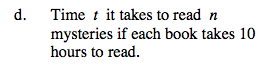t = 10n
Is this a proportional relationship?